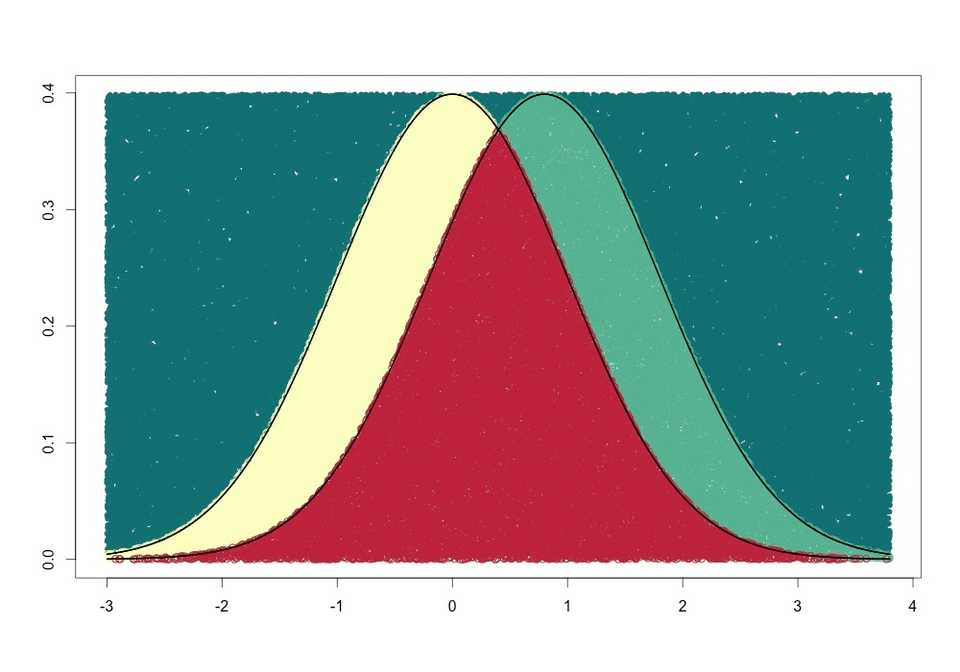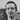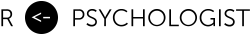# Calculating the Overlap of Two Normal Distributions Using Monte Carlo Integration

January 08, 2014

I read this post over at the blog Cartesian Faith about Probability and Monte Carlo methods. The post describe how to numerically intregate using Monte Carlo methods. I thought the results looked cool so I used the method to calculate the overlap of two normal distributions that are separated by a Cohen’s d of 0.8. You should head over to the original post if you want a more detailed explanation of the method. And I should add that this is not the most efficient way to calculate the overlap of two gaussian distributions, but it is a fun and pretty intuitive way, plus you can make a cool plot of the result. However, I also show how to get the overlap using the cumulative distribution function and using R’s built-in integration function.

## Overlapping proportions of two normal distributions

So two gaussian distributions that are separated by a standardized mean difference (Cohen’s d) of 0.8 look like thisTo calculate the overlap we just divide the number of points in the overlap region with the total numbers of points in one of the distributions. To get more stable results I calculate the mean overlap using both distributions. What we’re calculating is sometimes called the overlapping coefficient (OVL).

## The faster but less cool way

If we just want to convert from Cohen’s d to OVL, we can use the cumulative distribution function pnorm().

This result is very close to our monte carlo estimate. Another easy way is to use R’s built-in integrate() function, which will work with unequal variances as well.Written by Kristoffer Magnusson a researcher in clinical psychology. You should follow him on Twitter and come hang out on the open science discord Git Gud Science.

Share:

Published January 08, 2014 (View on GitHub)